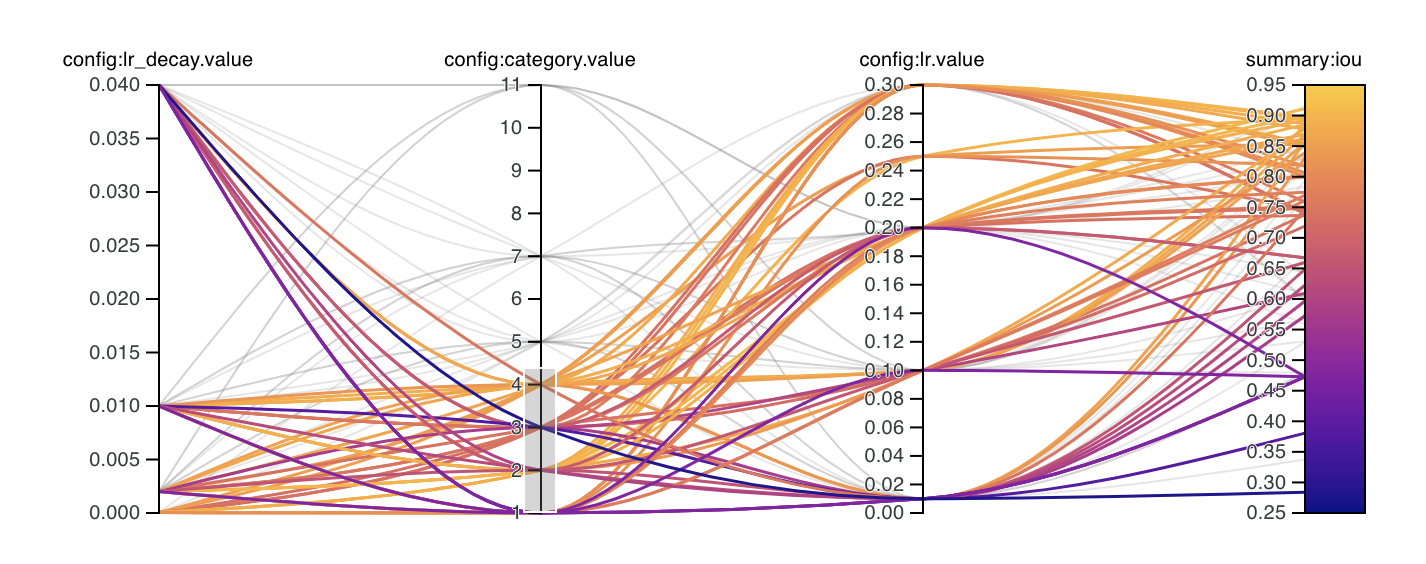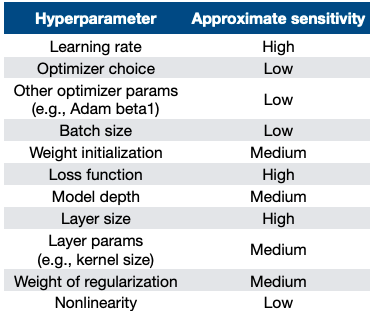# Running Hyperparameter Sweeps to Pick the Best ModelSayak Paul
16
Oct
2019

Hyperparameters play a crucial role in determining a machine learning model’s performance. In this post we’ll talk about searching through the hyperparameter space and finding the optimal model using sweeps from Weights & Biases.

Some people use parameters and hyperparameters interchangeably. Before we get started, it’s important that we explicitly call out the difference between the two:

• Parameters are not explicitly specified by a developer. Instead they are approximated and learned by a machine learning model. Weights and biases are the most granular parameters when it comes to neural networks.
• Hyperparameters are explicitly specified by a developer. In a neural network, examples of hyperparameters include the number of epochs, batch size, number of layers, number of nodes in each layer, and so on.Parallel Coordinates Plot Visualizing the Performance of Multiple Runs Over a Set Number of Hyperparameters

Most of a machine learning model’s performance is driven by hyperparameters. For example with a large dataset, if you train your neural network with a small number of epochs, it is very likely to underfit the data. So you might begin to ask yourself questions like:

• What should the ideal number of epochs be?
• What batch size should I use?
• How many layers should there be in the network?
• How many nodes should be in one layer?

All of these questions collectively lead us to: what model architecture should be used for the given dataset?

Google researchers address this in their seminal work on Neural Architecture Search (NAS), but one challenge stands out: NAS does not scale well.  NAS is computationally expensive, and many organizations do not have the bandwidth to support it.

However, there are ways to work around this:

• Specify the number of epochs that is close enough to the ideal one.
• Train multiple neural networks with a varying number of epochs and then come to a conclusion

The first takes quite a bit of deliberate practice and may not always produce the expected or even accurate result. That is why the latter is heavily used today. In machine learning literature, the process of experimenting with different hyperparameter values to select the best model is referred to as hyperparameter tuning. The following are very popular methods for hyperparameter tuning:

• Grid search
• Random search
• Bayesian optimization
• Hyperband

This brings us to Hyperparameter Sweeps – a way to efficiently select the right model for a given dataset using Weights & Biases (wandb).

## What are Hyperparameter Sweeps?

Hyperparameter Sweeps offer efficient ways of automatically finding the best possible combination of hyperparameter values for your machine learning model with respect to a particular dataset.

Conducting hyperparameter search is not a trivial task since not all  hyperparameters in a machine learning model have an equal priority when it comes to tuning them. Josh Tobin, in his Troubleshooting Deep Neural Networks presentation, has beautifully listed the hyperparameters in a typical neural network along with their priorities:Typical Hyperparameters in Neural Network Architecture - Source

Hyperparameter Sweeps organize search in a very elegant way, allowing us to:

• Set up hyperparameter searches using declarative configurations
• Experiment with a variety of hyperparameter tuning methods including grid search, random search, Bayesian optimization, and Hyperband

## Running Hyperparameter Sweeps using Weights & Biases

Weights & Biases makes it really easy to run Hyperparameter Sweeps. To start, you need a model training script (more on that shortly) and a dataset. You can use this Colab notebook if you want to follow along without working in the code directly. If you want to take a more active role set up wandb. If you have not, be sure to check this out. We will also use TensorFlow 2.0, specifically its high-level Keras API.

To start, let’s load up the FashionMNIST dataset that ships with Keras.

# Load the dataset

fashion_mnist = tf.keras.datasets.fashion_mnist
(train_images, train_labels), (test_images, test_labels) = fashion_mnist.load_data()
# Scale the pixel values of the images
train_images = train_images / 255.0
test_images = test_images / 255.0
# Select the hyperparameters you want to tune. This is specified like the following:
sweep_config = {
'method': 'grid',
'parameters': {
'layers': {
'values': [32, 64, 96, 128, 256]
}
}
}

Where method is the hyperparameter tuning method we are going for and parameters is a dictionary containing the hyperparameters we want to tune.

# Next, we initialize this sweep by running:

sweep_id = wandb.sweep(sweep_config)

When you run this, you should get a link to the sweep which you can view in the browser and use to track your sweep runs.

Once you have initialized the sweep you need an agent. An agent is a model training script you can use to pair the sweep configurations. Let’s define a simple training script:

def train():
# Initialize wandb with a sample project name
wandb.init(project="hyperparameter-sweeps-partI")

(X_train, y_train) = train_images, train_labels
(X_test, y_test) = test_images, test_labels

# Specify the hyperparameter to be tuned along with
# an initial value
configs = {
'layers': 128
}

# Specify the other hyperparameters to the configuration
config = wandb.config
config.epochs = 5

# Define the model
model = tf.keras.Sequential([
tf.keras.layers.Flatten(input_shape=(28, 28)),
tf.keras.layers.Dense(wandb.config.layers, activation=tf.nn.relu),
tf.keras.layers.Dense(10, activation=tf.nn.softmax)
])

# Compile the model
model.compile(optimizer='adam',
loss='sparse_categorical_crossentropy',
metrics=['accuracy'])

# Train the model
model.fit(X_train, y_train, epochs=config.epochs,
validation_data=(X_test, y_test),
callbacks=[WandbCallback(data_type="image", labels=labels)])

It’s super important to call wandb.init() at the beginning of the training script itself; otherwise, the different runs in the hyperparameter sweep will not be able to log the specified outputs to your wandb dashboard.

As you can see, we are trying to train a shallow fully-connected network. We are allowing the network to train for 5 epochs with the Adam optimizer (set to minimize the Sparse Category Crossentropy loss as the class labels are integers). This method is based on this example.

Once the model training script is done, you can run it as an agent to start the hyperparameter sweeping process.

wandb.agent(sweep_id, function=train)

And that’s it! You should see something like this in your notebook:

If you click on the sweep URL you got from the third step as described above, you should see a number of interesting things.

First, a dashboard giving you a summary of all the different runs (i.e. the network’s performance with a different set of layers) like so:

Note each run has its unique ID (left).

• Now if you click on any of the runs (that you can see on the left), you will be able to see the network’s performance individually:

Along with the network’s performance details, for each run, you also get:

• Network architecture
• A serialized version of the network in .h5 format
• Stats on bandwidth usage, CPU/GPU usage, memory footprint

Just click on a run of your choice, and you will have these buttons available on

the left:

While choosing the best model from the sweep we focus on the trade-off between the validation loss and training loss, i.e. we find the runs for which the validation loss kept on decreasing with respect to the training loss. The following table can come in really handy here.

On your Weights and Biases project page (example here), you can press 'option+space' to expand the runs table, and compare all the results. From here you can sort by validation accuracy (or validation loss) to find the model that performed best. You can also explore the results of the sweep by adding a parallel coordinates plot.

## How to Add a Parallel Coordinates Chart

Step 1: Click ‘Add visualization’ on the project page.

Step 2: Choose the parallel coordinates plot.

Step 3: Pick the dimensions (hyperparameters) you would like to visualize. Ideally, you want the last column to be the metric you are optimizing for (e.g. validation loss).

Step 4: And voila! You have a parallel coordinates plot!

In this plot, for example, we can see that a lower learning rate and a higher number of channels in our convolutional layers leads to a better validation accuracy. Parallel coordinate plots can be extremely useful in quickly seeing the trends in our hyperparameter values compared to our optimization metric. This, in turn, lets us narrow down our hyperparameter space and get to better accuracy faster.

If you’d like to learn more about how to choose the best model, check out these articles:

## Conclusion

Now that you understand how to use sweeps, I encourage you to try sweeps with multiple hyperparameters. In this tutorial, we only tuned the layers hyperparameter; you could also try tuning the batch_size, epochs, optimizer and so on.

I hope you enjoyed reading this tutorial. Stay tuned for the second part where we dive deeper with more advanced hyperparameter sweeps and many practical tips of using them effectively.

Join our mailing list to get the latest machine learning updates.